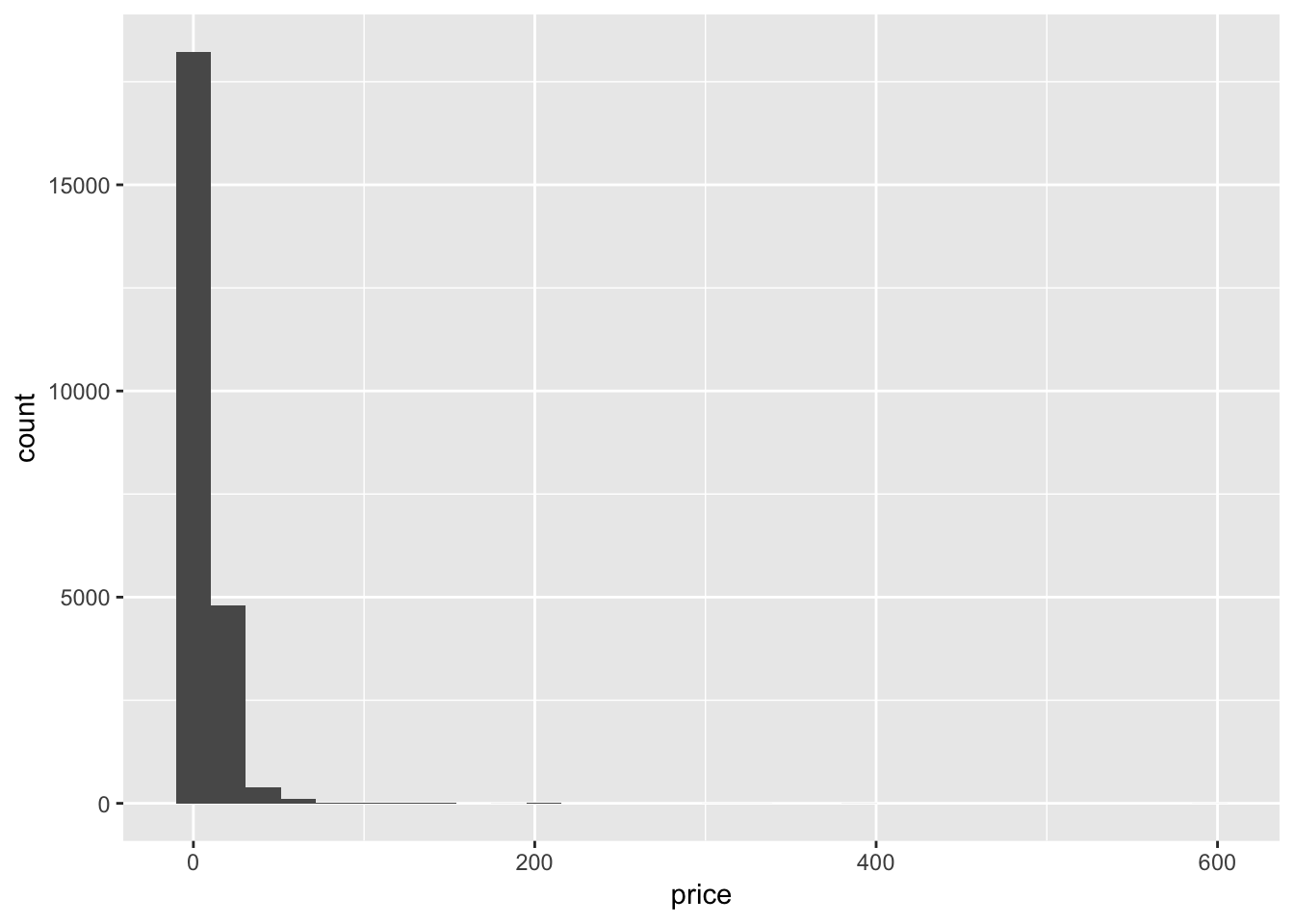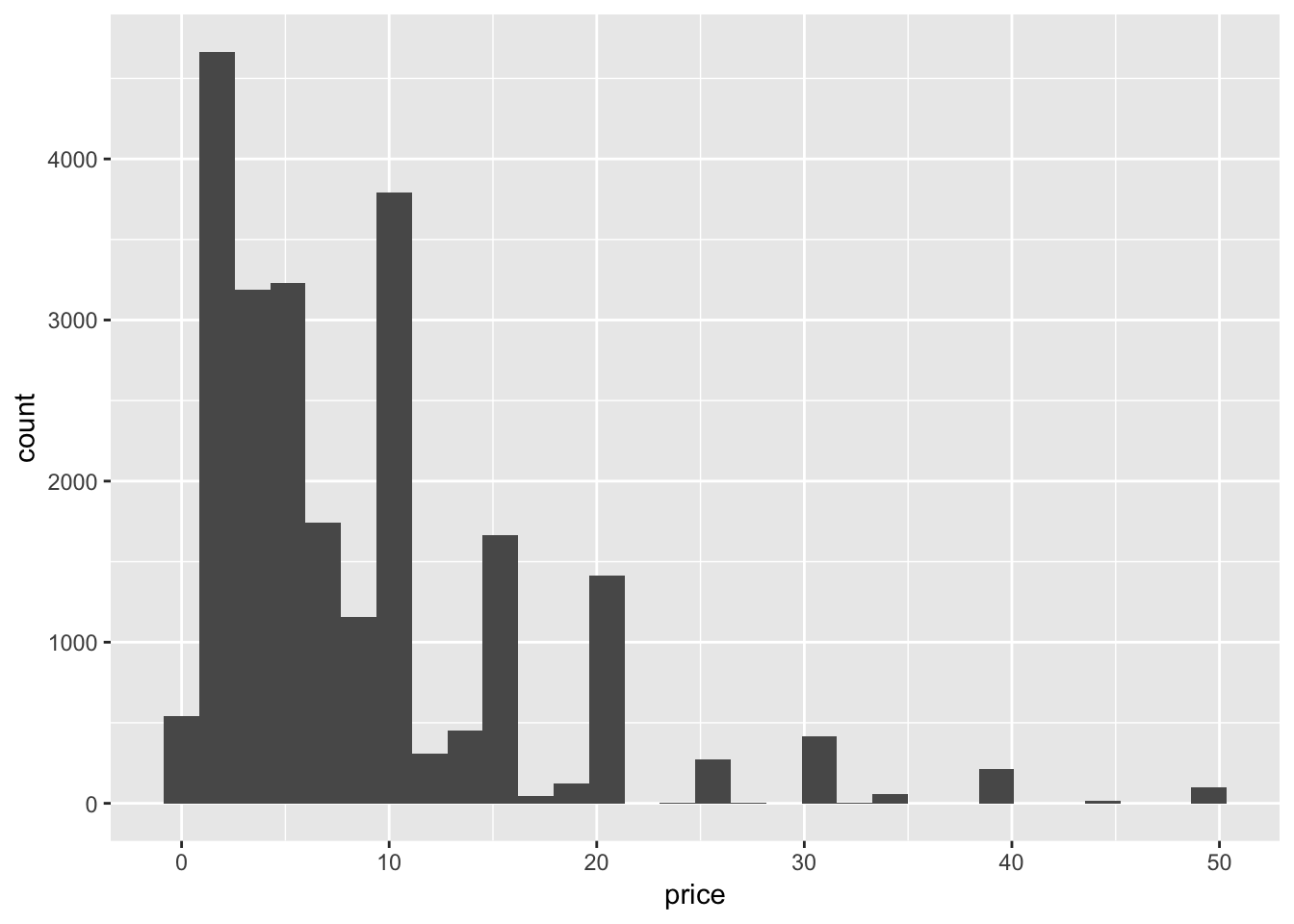## Background

https://github.com/rfordatascience/tidytuesday/tree/master/data/2019/2019-07-30

## Let’s Play!

library(tidyverse)
## ── Attaching packages ────────────────────────────────────────── tidyverse 1.2.1 ──
## ✔ ggplot2 3.1.0     ✔ purrr   0.2.5
## ✔ tibble  1.4.2     ✔ dplyr   0.7.7
## ✔ tidyr   0.8.1     ✔ stringr 1.4.0
## ✔ readr   1.1.1     ✔ forcats 0.3.0
## Warning: package 'stringr' was built under R version 3.5.2
## ── Conflicts ───────────────────────────────────────────── tidyverse_conflicts() ──
## ✖ dplyr::filter() masks stats::filter()
## ✖ dplyr::lag()    masks stats::lag()
# clean dataset from lizawood's github
url <- "https://raw.githubusercontent.com/lizawood/apps-and-games/master/PC_Games/PCgames_2004_2018_raw.csv"

# read in raw data
raw_df <- url %>%
read_csv() %>%
janitor::clean_names() 
## Parsed with column specification:
## cols(
##   # = col_integer(),
##   Game = col_character(),
##   Release date = col_character(),
##   Price = col_character(),
##   Score rank(Userscore / Metascore) = col_character(),
##   Owners = col_character(),
##   Playtime (Median) = col_character(),
##   Developer(s) = col_character(),
##   Publisher(s) = col_character()
## )
# clean up some of the factors and playtime data
clean_df <- raw_df %>%
mutate(price = as.numeric(price),
score_rank = word(score_rank_userscore_metascore, 1),
average_playtime = word(playtime_median, 1),
median_playtime = word(playtime_median, 2),
median_playtime = str_remove(median_playtime, "\$$"), median_playtime = str_remove(median_playtime, "\$$"),
average_playtime = 60 * as.numeric(str_sub(average_playtime, 1, 2)) +
as.numeric(str_sub(average_playtime, 4, 5)),
median_playtime = 60 * as.numeric(str_sub(median_playtime, 1, 2)) +
as.numeric(str_sub(median_playtime, 4, 5)),
metascore = as.double(str_sub(score_rank_userscore_metascore, start = -4, end = -3))) %>%
select(-score_rank_userscore_metascore, -score_rank, -playtime_median) %>%
rename(publisher = publisher_s, developer = developer_s)
## Warning in evalq(as.numeric(price), <environment>): NAs introduced by
## coercion
## Warning in evalq(60 * as.numeric(str_sub(average_playtime, 1, 2)) +
## as.numeric(str_sub(average_playtime, : NAs introduced by coercion
## Warning in evalq(60 * as.numeric(str_sub(median_playtime, 1, 2)) +
## as.numeric(str_sub(median_playtime, : NAs introduced by coercion
## Warning in evalq(as.double(str_sub(score_rank_userscore_metascore, start =
## -4, : NAs introduced by coercion

## Explore

Let’s see how price is spread out over the dataset.

clean_df %>%
ggplot(aes(x=price)) +
geom_histogram()
## stat_bin() using bins = 30. Pick better value with binwidth.
## Warning: Removed 3095 rows containing non-finite values (stat_bin).Looks like there are some “video games” that are super expensive. But there aren’t that many.

clean_df %>%
select(game, price) %>%
filter(price > 100) %>%
summarise(count=n())
## # A tibble: 1 x 1
##   count
##   <int>
## 1    27

Let’s narrow in on where most of the data is.

clean_df %>%
select(game, price) %>%
filter(price < 50) %>%
ggplot(aes(price)) +
geom_histogram()
## stat_bin() using bins = 30. Pick better value with binwidth.There are spikes around the even-numbered price points (like 19.99 or 9.99).

## How much do different developers sell their games for?

clean_df %>%
na.omit() %>%
select(price, game, developer) %>%
group_by(developer) %>%
summarise(average_cost = mean(price), game_count = n())
## # A tibble: 1,767 x 3
##    developer                    average_cost game_count
##    <chr>                               <dbl>      <int>
##  1 [bracket]games                       4.99          1
##  2 ][ Games Inc                        15.0           1
##  3 @unepic_fran                        13.0           1
##  4 10tons Ltd                          14.7           4
##  5 11 bit studios                      17.0           5
##  6 11Sheep                              8.99          1
##  7 14° East                             9.99          1
##  8 17-BIT                              15.0           2
##  9 1C Entertainment                     5.56          7
## 10 1C Game Studios, 777 Studios        50.0           1
## # ... with 1,757 more rows

Looks like a lot of these developers only have put out one or two games. Maybe we can look at the publishers.

game_costs <- clean_df %>%
na.omit() %>%
select(price, game, publisher) %>%
group_by(publisher) %>%
summarise(average_cost = mean(price), game_count = n()) %>%
arrange(desc(game_count))
game_costs %>%
head()
## # A tibble: 6 x 3
##   publisher           average_cost game_count
##   <chr>                      <dbl>      <int>
## 1 Ubisoft                     15.3         81
## 2 THQ Nordic                  15.7         53
## 3 Devolver Digital            14.0         47
## 4 Square Enix                 14.2         44
## 5 Paradox Interactive         18.9         43
## 6 SEGA                        21.1         40